RS Aggarwal Class 9 Solutions Chapter 7 - Areas Ex 7A (7.1)

RS Aggarwal Class 9 Chapter 7 - Areas Ex 7A (7.1) Solutions Free PDF

Q.1: The height of an equilateral triangle measures 9 cm. Find its area correct to 2 decimal places. (Take root 3 is 3=1.732$\sqrt{3}=1.732$)

Sol:

Let, a be the side of an equilateral triangle.

Therefore, the height of an equilateral triangle = 34×a$\frac{\sqrt{3}}{4}\times a$ units

Height of an equilateral triangle = 9cm (given)

32a=9$\Rightarrow \frac{\sqrt{3}}{2}a=9$
a=9×23$\Rightarrow a=\frac{9\times 2}{\sqrt{3}}$

a=9×2×33×3$\Rightarrow a=\frac{9\times 2\times \sqrt{3}}{\sqrt{3}\times \sqrt{3}}$ [rationalizing the denominator ]

9×2×33$\Rightarrow \frac{9\times 2\times \sqrt{3}}{3}$
a=63$\Rightarrow a=6 \sqrt{3}$
base=63$\Rightarrow base =6 \sqrt{3}$

Area of the equilateral triangle = 12×base×height$\frac{1}{2}\times base\times height$

= 12×63×9$\frac{1}{2}\times 6\sqrt{3} \times 9$ = 273$27\sqrt{3}$

Area of the equilateral triangle = 27×1.732=46.764$27\times 1.732 =46.764$ = 46.76 cm2

Q.2: An umbrella is made by stitching 12 triangular pieces of cloth, each measuring 50cm×20cm×50cm$50cm\times 20cm\times 50cm$. Find the area of the cloth used in it.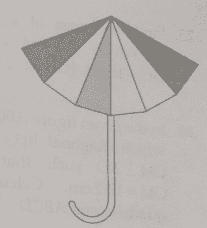Sol:

Let a = 50 cm, b = 20 cm and c = 50 cm

Now,

S = 12(a+b+c)$\frac{1}{2}(a+b+c)$

S = 12(50+20+50)=1202=60cm$\frac{1}{2}(50+20+50) \; =\frac{120}{2}= 60 \; cm$

Therefore, area of triangular piece of cloth =S(Sa)(Sb)(Sc)$\sqrt{S(S-a)(S-b)(S-c)}$

= 60(6050)(6020)(6050)$\sqrt{60(60-50)(60-20)(60-50)}$

= 60×10×40×10$\sqrt{60 \times 10 \times 40 \times 10 }$

= 6×10×10×4×10×10$\sqrt{6 \times 10 \times 10 \times 4 \times 10 \times 10 }$

= 10×10×10×10×2×2×2×3$\sqrt{ 10 \times 10 \times 10 \times 10 \times 2 \times 2 \times 2 \times 3 }$

= 10×10×26$10 \times 10 \times 2 \sqrt{6 }$

= 2006=200×2.45=490cm2$200 \sqrt{6 } = 200 \times 2.45 = 490 \; cm^{2}$

Therefore, the area of one piece of cloth = 490 cm2$cm^{2}$

Now, the area of 12 pieces = 12×490cm2=5880cm2$12 \times 490 \; cm^{2} = 5880 \; cm^{2}$

Q.3: A floral design on a floor is made up of 16 tiles, each triangular in shape having sides 16 cm, 12 cm and 20 cm. Find the cost of polishing the tiles at Re 1 per cm2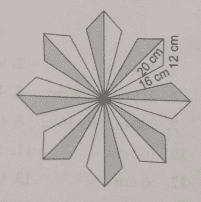Sol:

Let, a = 16 cm, b = 12 cm and c = 20 cm

Now,

S = 12(a+b+c)$\frac{1}{2}(a+b+c)$

S = 12(16+12+20)=482=24cm$\frac{1}{2}(16+12+20) \; =\frac{48}{2}= 24 \; cm$

Area of one triangular tile =S(Sa)(Sb)(Sc)$\sqrt{S(S-a)(S-b)(S-c)}$

= 24(2416)(2412)(2420)$\sqrt{24(24-16)(24-12)(24-20)}$

= 2×2×2×2××2×2×2×2×2×2×3×3$\sqrt{2 \times 2 \times 2 \times 2 \times \times 2 \times 2 \times 2 \times 2 \times 2 \times 2 \times 3 \times 3}$

= 2×2×2×2×2×3$\sqrt{2 \times 2 \times 2 \times 2 \times 2 \times 3 }$

Area of one tile = 96 cm2

$\Rightarrow$ area of all tiles = 96×16=1536cm2$96\times 16 =1536 \;cm^{2}$

Cost of polishing the tiles per sq. cm = Re. 1

Thus, the total cost of polishing all the tiles = Rs. (1×1536)=Rs.1536$1 \times 1536) = Rs. \; 1536$

Q.4: Find the perimeter and area of the quadrilateral ABCD in which AB = 17 cm, AD = 9 cm, CD = 12cm, ACB=90$\angle ACB=90^{\circ}$ and AC = 15 cm.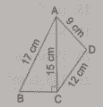Sol:

Consider the right triangle ABC.

Now, by Pythagoras theorem, we have

BC = AB2AC2$\sqrt{AB^{2}-AC^{2}}$ = 172152$\sqrt{17^{2}-15^{2}}$ = 289225$\sqrt{289-225}$

Therefore, BC = 64$\sqrt{64}$ = 8 cm

Now, the Perimeter of quad. ABCD = 17 + 9 + 12 + 8 = 46 cm

Area of triangle ABC=12×base×height$\bigtriangleup ABC = \frac{1}{2}\times base \times height$

ABC=12×8×15$\bigtriangleup ABC = \frac{1}{2}\times 8 \times 15$

= 60 cm2$cm^{2}$

For area of triangle, ACD:

Let, a = 15 cm, b = 12 cm and c = 9 cm

Now,

S = 12(a+b+c)$\frac{1}{2}(a+b+c)$

S = 12(15+12+9)=362=18cm$\frac{1}{2}(15+12+9) \; =\frac{36}{2}= 18 \; cm$

Area of one triangular tile  =S(Sa)(Sb)(Sc)$\sqrt{S(S-a)(S-b)(S-c)}$

=18(1815)(1812)(189)$=\sqrt{18(18-15)(18-12)(18-9)}$
=18×3×6×9$=\sqrt{18 \times 3 \times 6 \times 9}$
=18×18×3×3$=\sqrt{18 \times 18 \times 3 \times 3}$

= 18×3=54cm2$18 \times 3= 54 \; cm^{2}$

Thus the area of quadrilateral ABCD= area of ABC$\bigtriangleup ABC$ + area of ACD$\bigtriangleup ACD$

= 60 + 54 = 114 cm2

Q.5: Find the perimeter and area of the quadrilateral ABCD in which AB = 42cm, BC = 21cm, CD = 29cm, DA=34 cm and CBD=90$\angle CBD=90^{\circ}$.

Sol:

Perimeter of quad. ABCD = 34 + 29 + 21 + 42 = 126 cm

Area of triangle BCD = 12×base×height$\frac{1}{2}\times base \times height$

Area of triangle BCD = 12×20×21=210cm2$\frac{1}{2}\times 20 \times 21 =210 \; cm^{2}$

For area of triangle ABC,

Let, a = 42 cm, b = 20 cm and c = 34 cm

Now,

S = 12(a+b+c)$\frac{1}{2}(a+b+c)$

S = 12(42+20+34)=962=48cm$\frac{1}{2}(42+20+34) \; =\frac{96}{2}= 48 \; cm$

Area of triangle =S(Sa)(Sb)(Sc)$\sqrt{S(S-a)(S-b)(S-c)}$

= 48(4842)(4820)(4834)$\sqrt{48(48-42)(48-20)(48-34)}$

= 48×6×28×14$\sqrt{48 \times 6 \times 28 \times 14}$

= 16×3×3×2×2×14×14$\sqrt{16 \times 3 \times 3 \times 2 \times 2 \times 14 \times 14}$

=4×3×2×14=336cm2$4 \times 3 \times 2 \times 14 = 336 \; cm^{2}$

Area of quadrilateral ABCD = area of ABD$\bigtriangleup ABD$ + area of BCD$\bigtriangleup BCD$

Thus, the area of quadrilateral ABCD = 336 + 210 = 546 cm2

Q.6: Find the area of the quadrilateral ABCD in which AB = 24 cm, BAC=90$\angle BAC = 90^{\circ}$ and BCD$\bigtriangleup BCD$ is an equilateral triangle having each side equals to 26cm. Also, find the perimeter of the quadrilateral. (Given 3=1.73$\sqrt{3}=1.73$ )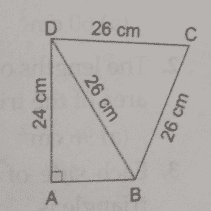Sol:

Consider the right triangle ABD,

By pythagoras theorem, we have

AB=BD2AD2$AB=\sqrt{BD^{2}-AD^{2}}$ = 262242$\sqrt{26^{2}-24^{2}}$ = 676576$\sqrt{676-576}$

Therefore, AB = 100$\sqrt{100}$ =10 cm

base=10cm$\Rightarrow base = 10 \; cm$

Area of the triangle ABD = 12×base×height$\frac{1}{2}\times base \times height$

$\Rightarrow$ area of ABD=12×10×24$\bigtriangleup ABD =\frac{1}{2}\times 10\times 24$

AreaofABD=120cm2$\Rightarrow \;Area \; of \;\bigtriangleup ABD =120 \; cm^{2}$

Area of equilateral triangle BCD = 34a2$\frac{\sqrt{3}}{4}a^{2}$

1.734(26)2$\frac{1.73}{4}(26)^{2}$ = 292.37 cm2

Area of quad. ABCD = area of ABD$\bigtriangleup ABD$ + area of BCD$\bigtriangleup BCD$

= 120 + 292.37 = 412.37 cm2

Q.7: Find the area of parallelogram ABCD in which AB = 28 cm, BC = 26cm and diagonal AC = 30 cm.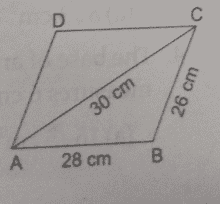Sol:

Consider the triangle ABC,

Let, a = 26 cm, b = 30 cm and c = 28 cm

Now,

S = 12(a+b+c)$\frac{1}{2}(a+b+c)$

S = 12(26+30+28)=842=42cm$\frac{1}{2}(26+30+28) \; =\frac{84}{2}= 42 \; cm$

Area of triangle ABC = S(Sa)(Sb)(Sc)$\sqrt{S(S-a)(S-b)(S-c)}$

= 42(4226)(4330)(4228)$\sqrt{42(42-26)(43-30)(42-28)}$

= 42×16×12×14$\sqrt{42 \times 16 \times 12 \times 14}$

= 14×3×16×4×3×14$\sqrt{14 \times 3 \times 16 \times 4 \times 3 \times 14}$

= 14×14×3×3×16×4$\sqrt{14 \times 14 \times 3 \times 3 \times 16 \times 4}$

= 4×3×2×14=336cm2$4 \times 3 \times 2 \times 14 = 336 \; cm^{2}$

In a parallelogram, diagonal divides the parallelogram in two equal area

Therefore area of quadrilateral ABCD= area of ABC$\bigtriangleup ABC$ + area of ACD$\bigtriangleup ACD$

= Area of area of ABC×2$\bigtriangleup ABC \times 2$

= 336 ×2$\times 2$ = 672 cm2

Q.8: Find area of parallelogram ABCD in which AB = 14 cm, BC = 10cm and AC = 16 cm. (Given 3=1.73$\sqrt{3}=1.73$)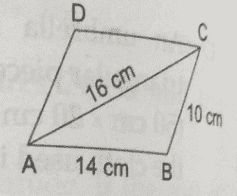Sol:

Consider the triangle ABC,

Let, a = 10 cm, b = 16 cm and c = 14 cm

S = 12(a+b+c)$\frac{1}{2}(a+b+c)$

S = 12(10+16+14)=402=20cm$\frac{1}{2}(10+16+14) \; =\frac{40}{2}= 20 \; cm$

Area of triangle ABC = S(Sa)(Sb)(Sc)$\sqrt{S(S-a)(S-b)(S-c)}$

= 20(2010)(2016)(2014)$\sqrt{20(20-10)(20-16)(20-14)}$

= 20×10×4×6$\sqrt{20 \times 10 \times 4 \times 6}$

= 10×2×10×4×3×2$\sqrt{10 \times 2 \times 10 \times 4 \times 3 \times 2}$

= 14×10×4×2×2×2×3$\sqrt{14 \times 10 \times 4 \times 2 \times 2 \times 2 \times 3}$

= 10×2×2×3=403cm2$10 \times 2 \times 2 \times \sqrt{3} = 40 \sqrt{3} \; cm^{2}$

In a parallelogram, diagonal divides the parallelogram in two equal areas

Therefore, the area of quadrilateral ABCD= area of ABC$\bigtriangleup ABC$ + area of ACD$\bigtriangleup ACD$

= Area of area of ABC×2$\bigtriangleup ABC \times 2$

= 403×2cm2$40 \sqrt{3}\times 2 \; cm^{2}$

= 803cm2$80 \sqrt{3} \; cm^{2}$ = 138.4 cm2

Q.9: In the given figure ABCD is a quadrilateral in which diagonal BD =64 cm ALBD$AL\perp BD$ and CMBD$CM\perp BD$ such that AL= 16cm and CM =16.8 cm. Calculate the area of quadrilateral ABCD.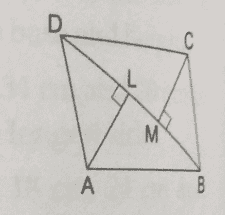Sol:

Area of triangle ABD = 12×base×height$\frac{1}{2}\times base \times height$

= 12×BL×AL$\frac{1}{2}\times BL \times AL$

= 12×64×16.8$\frac{1}{2}\times 64 \times 16.8$ = 537.6 cm2

Area of triangle BCD = 12×base×height$\frac{1}{2}\times base \times height$

= 12×BD×CM$\frac{1}{2}\times BD \times CM$

= 12×64×13.2$\frac{1}{2}\times 64 \times 13.2$ = 422.4 cm2

Therefore, the area of quadrilateral ABCD= Area of ABD$\bigtriangleup ABD$ + BCD$\bigtriangleup BCD$ = 537.6 + 422.4 = 960 cm2

Practise This Question

Match Column - I with Column - II and select the correct answer using the codes given below the columns.

Column - I (Organelles)Column - II (Membranes)A. MitochondriaP. De DuveB. GolgiQ. Camello golgiC. NucleusR. BendaD. LysosomesS. Robert Brown